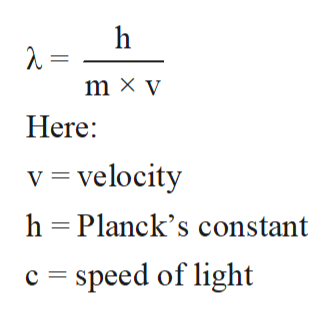The velocity of the electron in the ground state of the hydrogen atom is 3.10×106 m/s. Hint: take a look at the De Brogile equation. Also,                  1 J = 1(kg•m2) / s2.What is the wavelength of this electron in meters?

Question

The velocity of the electron in the ground state of the hydrogen atom is 3.10×106 m/s. Hint: take a look at the De Brogile equation. Also,                  1 J = 1(kg•m2) / s2.

What is the wavelength of this electron in meters?

Step 1

Bohr’s theory of atoms was applicable to the one electron system. It purposed the energy levels in an atom. It purposed the spectrum of atoms with lines for each electronic transition in an atom.

Step 2

The de Broglie equation purposed the relation between wavelength and mass of the photon. The mathematical expression to this relation is:help_outlineImage Transcriptioncloseh m x y Here: v velocity h Planck's constant speed of light c fullscreen
Step 3

Velocity of electron = 3.10×106 m/s.

Mass of electron =9.1 × 10-31 kilograms

h = Planck&rs...

Want to see the full answer?

See Solution

Want to see this answer and more?

Our solutions are written by experts, many with advanced degrees, and available 24/7

See Solution
Tagged in

Chemistry